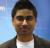How to display hour:minute:second values in charts?

Recommended Posts

Hello,

How to display time-duration values in charts?

Example value :  00:00:02:88 (2 seconds, 88 mili-seconds).

We must see 2 digits for the hours, minutes and seconds, and three digits for the mili-seconds.

Best Regards,

Ofer Frohlich

Share on other sites

Which version is it?

Share on other sites

Is it possible with FusionCharts v.3 ?

Share on other sites
Pallav (2/27/2007)
I'm afraid it's not currently possible with FusionCharts.

So how does one create charts where the y axis is a duration?

Share on other sites

i don't want use this sample:

http://docs.fusionch...um_Scaling.html

```<chart defaultNumberScale='s' numberScaleValue='60,60,24,7' numberScaleUnit='min,hr,day,wk'>
<set label='A' value='38' />
<set label='B' value='150' />
<set label='C' value='81050' />
<set label='D' value='334345' />
<set label='E' value='534345' />
</chart>```

because i like see 150 -> 2min. 30 sec.

no 2,5 min

It's possible use an ALIAS TAG to force the conversion?

For example:

```<chart >
<set label='A' value='38' alias='38 sec' />
<set label='B' value='150' alias='2 min. 30 sec' />
</chart>```

Edited by Janka74

Share on other sitesi don't want use this sample:

http://docs.fusionch...um_Scaling.html

```<chart defaultNumberScale='s' numberScaleValue='60,60,24,7' numberScaleUnit='min,hr,day,wk'>
<set label='A' value='38' />
<set label='B' value='150' />
<set label='C' value='81050' />
<set label='D' value='334345' />
<set label='E' value='534345' />
</chart>```

because i like see 150 -> 2min. 30 sec.

no 2,5 min

It's possible use an ALIAS TAG to force the conversion?

For example:

```<chart >
<set label='A' value='38' alias='38 sec' />
<set label='B' value='150' alias='2 min. 30 sec' />
</chart>```

Hi,

You can use dsiplayValue attribute for displaying custom value.

```<chart >
<set label='A' value='38' displayValue='38 sec' />
<set label='B' value='150' displayValue='2 min. 30 sec' />
</chart>```